Mathematics
Easy

Question

# Rewrite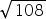into simplest form

##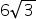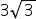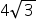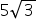Hint:

## The correct answer is:### Here, we have to write the simplest form of √108 .Now, √108 = √ ( 2 × 2 × 3 × 3 × 3 )= √ ( 22 × 32 × 3 )= ( 2 × 3 ) √3= 6√3Hence, the correct option is (a) .

The square root is an inverse method lf squaring a number.

### Related Questions to study#### With Turito Foundation.#### Get an Expert Advice From Turito.